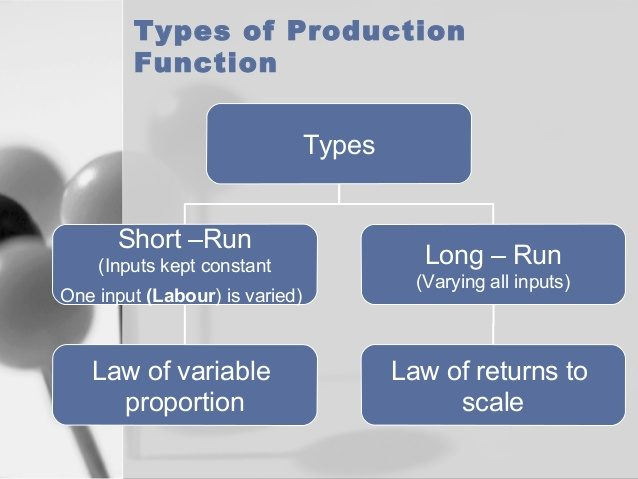# AGRIC6: Production Functions: Meaning and Types

##### The unit explains what production function means, it also looks at the types of Production Functions and Laws of Returns: Increasing, Constant and Decreasing

The production function portrays an input-output relationship. It describes the rate at which resources are transformed into products. There are numerous input-output relationships in agriculture because the rates at which the inputs are transformed into outputs will vary among soil types, animals, technologies, rainfall amount and so forth.Definition: Production function is a technical and mathematical relationship describing the manner and extent to which a particular product depends upon the quantities of inputs or services of inputs, used at a given level of technology and in a given period of time. It shows the quantity of output that can be produced using different levels of inputs.

It refers to the relationship between the input of factor services and the output of the resultant product. The production function is based on the idea that the amount of output in a production process depends upon the amount of inputs used in the process

A production function can be expressed in different ways: in written form, enumerating and describing the inputs that have a bearing on the output; by listing inputs and the resulting outputs numerically in a table; depicting in the form of a graph or a diagram; and in the form of an algebraic equation. Symbolically, a production function can be written as

Y=f (X1, X2 , X3 ,…….., Xn) where Y is output, X1, X2 , X3….. Xn are inputs. It, however, does not tell which inputs are fixed and which are the variable ones. Since in production, fixed inputs play an important role, these are expressed as: Y=f (X1, X2 / X3…..Xn) where Y is output, X1, X2 are variable inputs and X3…..Xn are fixed inputs.

Assumptions of Production Function Analysis
1. The production function is defined only for the non-negative values of inputs and outputs.
2. The production function presupposes technical efficiency. This means that every possible combination of inputs is assumed to result in maximum level of output.
3. The input- output relationship or the production function is single valued and continuous.
4. The production function is characterized by i) decreasing marginal product for all factor- product combinations; ii) decreasing rate of technical substitution between any two factors; and iii) an increasing rate of product transformation between any two products.
5. The returns to scale are assumed to be decreasing.
6. All the factors of production and products are perfectly divisible.
7. The parameters determining the firm’s production function do not change over the time period considered. Also, these parameters are not allowed to be random variables.

8. The exact nature of any production function is assumed to be determined by a set of technical decisions taken by the producer.

### Types of Production Functions

Several types of production functions used in agriculture are as follows:

1. Linear Production Function: Also known as first degree polynomial. It’s algebraic form is given by

y = a0 + bx where a0 is the intercept and b is the slope of the function. It is not commonly used in research because it violates the basic assumptions of characteristic functional analysis.

## Laws of Returns: Increasing, Constant and Decreasing

In production one or a combination of the following relationships are commonly observed:

1. Law of constant marginal returns (productivity),
2. Law of increasing marginal returns (productivity) and
3. Law of decreasing marginal returns (productivity)
1. Law of constant marginal returns (productivity): It is said to operate when each marginal unit of variable input adds equal quantity of output to the total output. It is applicable over limited range, e.g. one tractor (plus driver) will almost give same output, other things remaining constant.

 Fertilizer (X)(in kg) Total Product (Y)(in kg) Marginal Product (Returns)(ΔY/Δ X) 0 1300 – 10 1350 5 20 1400 5 30 1450 5 40 1500 5 50 1550 5

Algebraically, ΔY1/ΔX1 = ΔY2/ΔX2 =……………..= ΔYn/ΔXn

1. Law of increasing marginal returns (productivity): It is said to operate when each marginal unit of variable input adds more and more quantity of output to the total output. It is not common in agriculture, e.g. small increase in seed input given the fixed

 Seed (X) (inkg) Total Product (Y)(in kg) Marginal Product (Returns)(ΔY/Δ X) 10 1000 – 15 1025 5 20 1075 10 25 1150 15 30 1250 20 35 1375 25

Algebraically, ΔY1/ΔX1 < ΔY2/ΔX2 <……………..< ΔYn/ΔXn

1. Law of decreasing marginal returns (productivity): It is said to operate when each marginal unit of variable input adds less and less quantity of output to the total output. It is widely applicable in

 Fertilizer (X) (in kg) Total Product (Y) (in kg) Marginal Product (Returns) (ΔY/Δ X) 0 500 – 10 1400 90 20 2100 70 30 2600 50 40 3000 40 50 3300 30 60 3500 20

Algebraically, ΔY1/ΔX1 > ΔY2/ΔX2 >……………..> ΔYn/ΔXn

SEE ALL
•YOU